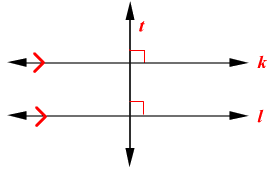# Perpendicular Transversal Theorem

In a plane, if a line is perpendicular to one of two parallel lines , then it is perpendicular to the other line also.Given:

$k\parallel l,\text{\hspace{0.17em}}\text{\hspace{0.17em}}t\perp k$

Prove:

$t\perp l$

Proof

 Statement Reason 1 $k\parallel l,\text{\hspace{0.17em}}\text{\hspace{0.17em}}t\perp k$ Given 2 Let the angle between the lines $t$ and $k$ be $1$ and that between $t$ and $l$ be $2$ . $\angle 1$ is a right angle Definition of perpendicular  lines 3 $m\angle 1=90°$ Definition of right angle 4 $\angle 1\cong \angle 2$ Corresponding angles 5 $m\angle 1=m\angle 2$ Definition of congruent angles 6 $m\angle 2=90°$ Substitution Property 7 $\angle 2$ is a right angle Definition of right angle 8 $t\perp l$ Definition of perpendicular lines